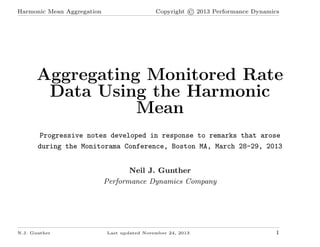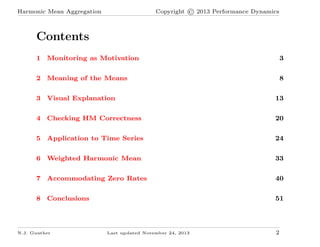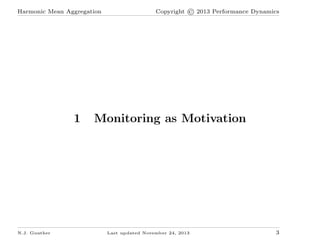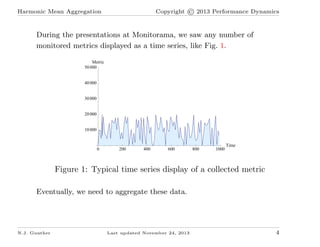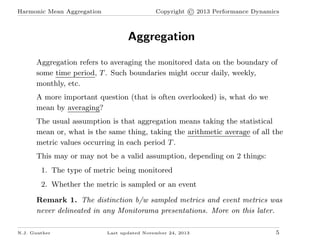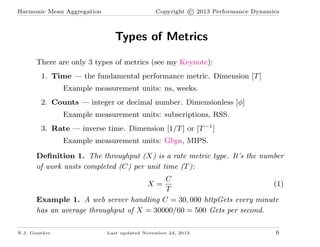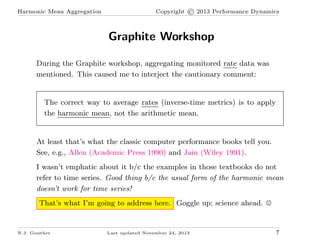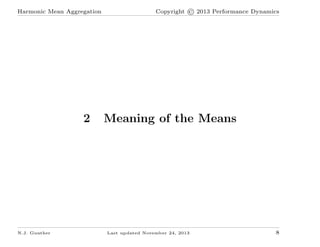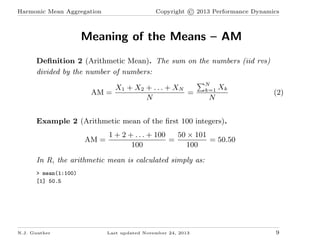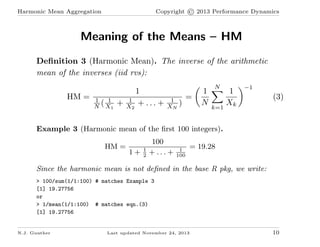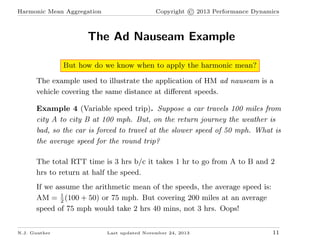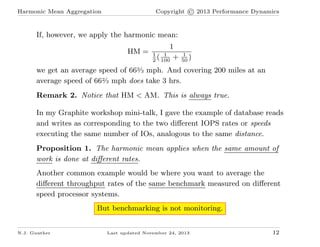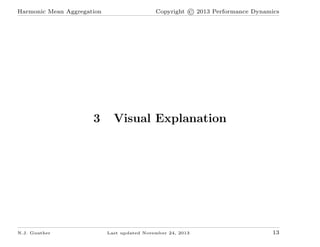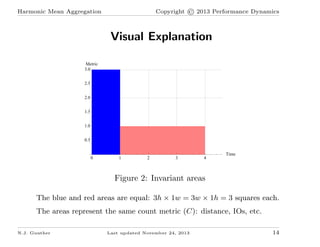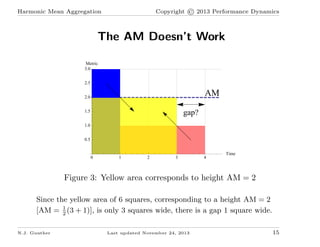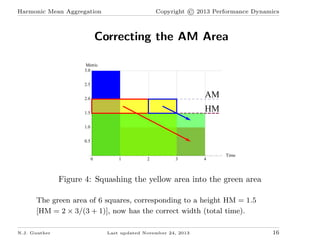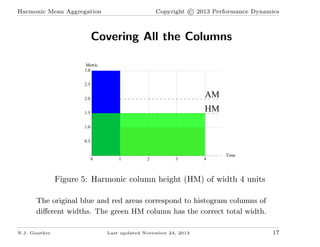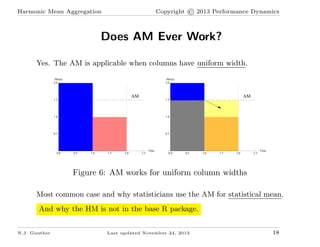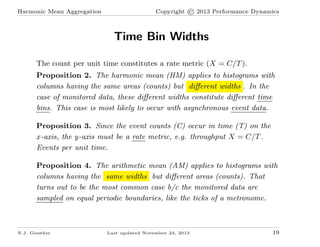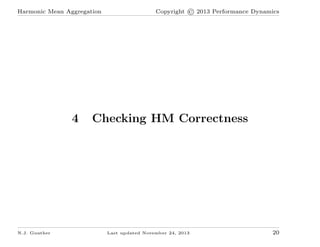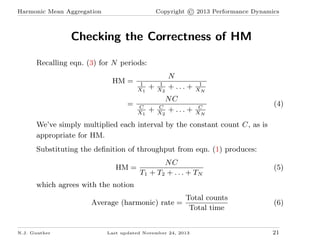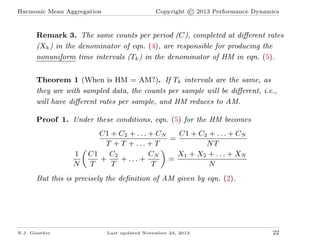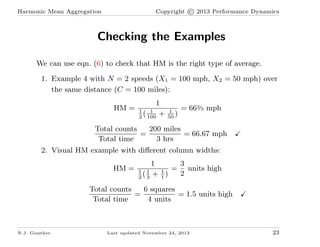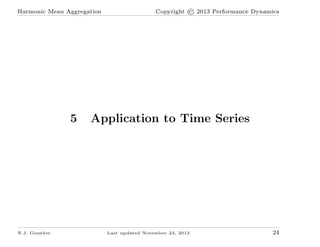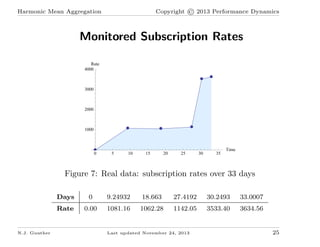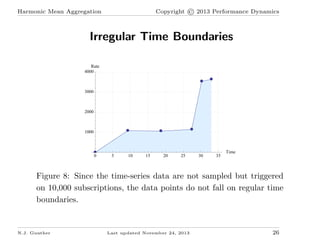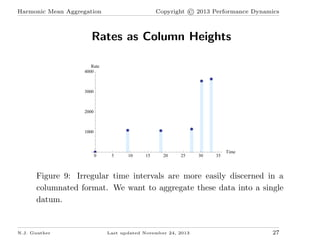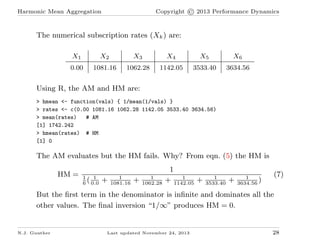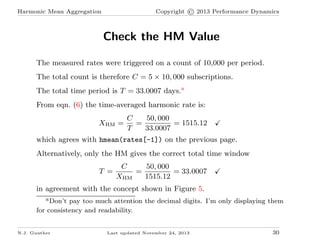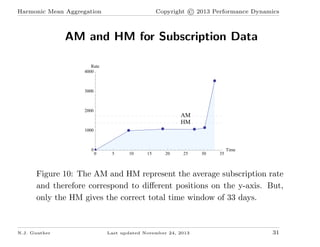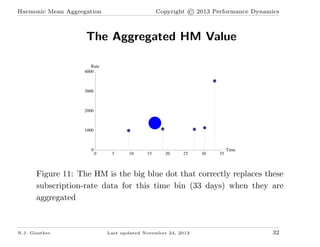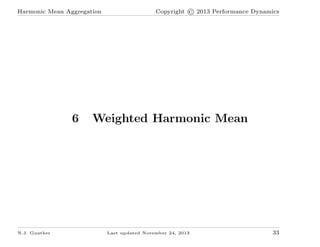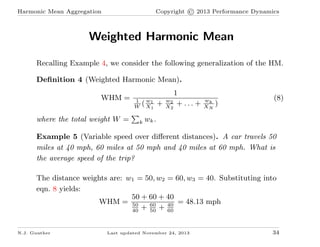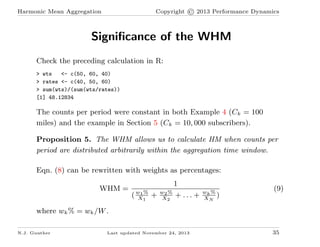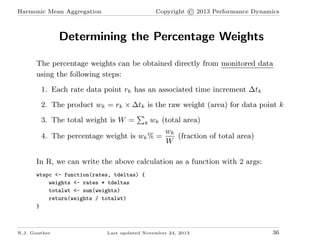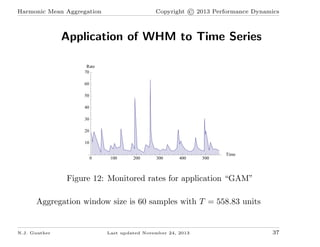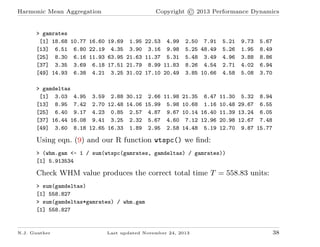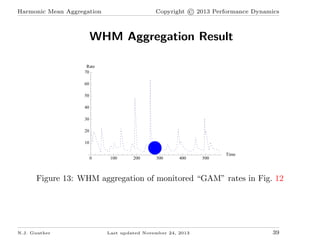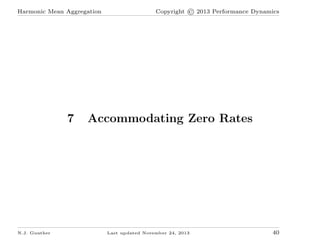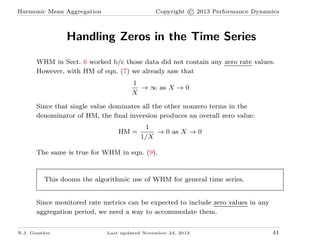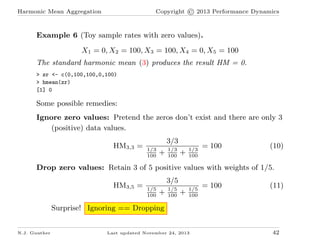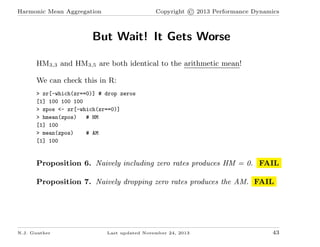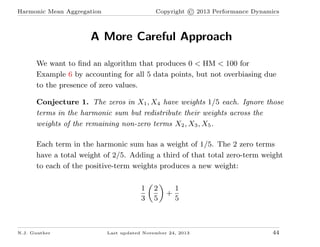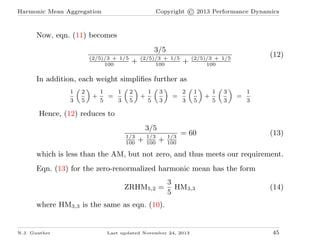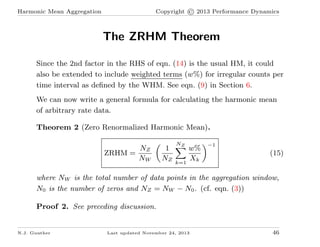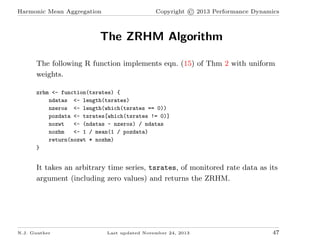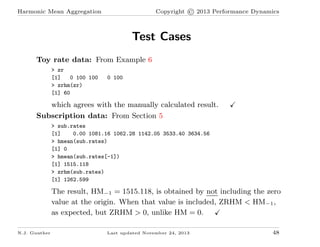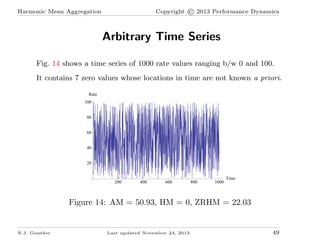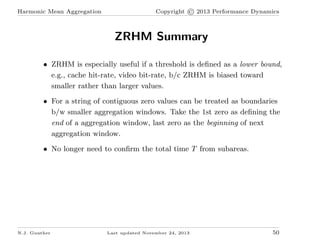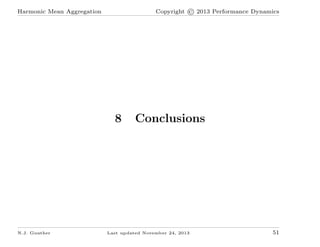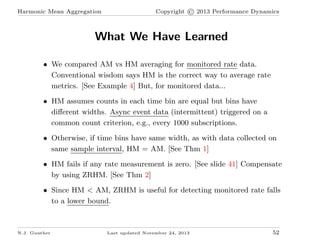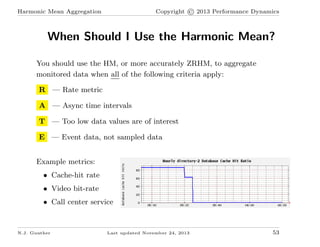1 of 53

### Harmonic Mean for Monitored Rate Data

1. Harmonic Mean Aggregation Copyright © 2013 Performance Dynamics Aggregating Monitored Rate Data Using the Harmonic Mean Progressive notes developed in response to remarks that arose during the Monitorama Conference, Boston MA, March 28-29, 2013 Neil J. Gunther Performance Dynamics Company N.J. Gunther Last updated November 24, 2013 1
2. Copyright © 2013 Performance Dynamics Harmonic Mean Aggregation Contents 1 Monitoring as Motivation 3 2 Meaning of the Means 8 3 Visual Explanation 13 4 Checking HM Correctness 20 5 Application to Time Series 24 6 Weighted Harmonic Mean 33 7 Accommodating Zero Rates 40 8 Conclusions 51 N.J. Gunther Last updated November 24, 2013 2
3. Harmonic Mean Aggregation 1 N.J. Gunther Copyright © 2013 Performance Dynamics Monitoring as Motivation Last updated November 24, 2013 3
4. Copyright © 2013 Performance Dynamics Harmonic Mean Aggregation During the presentations at Monitorama, we saw any number of monitored metrics displayed as a time series, like Fig. 1. Metric 50 000 40 000 30 000 20 000 10 000 0 200 400 600 800 1000 Time Figure 1: Typical time series display of a collected metric Eventually, we need to aggregate these data. N.J. Gunther Last updated November 24, 2013 4
5. Harmonic Mean Aggregation Copyright © 2013 Performance Dynamics Aggregation Aggregation refers to averaging the monitored data on the boundary of some time period, T . Such boundaries might occur daily, weekly, monthly, etc. A more important question (that is often overlooked) is, what do we mean by averaging? The usual assumption is that aggregation means taking the statistical mean or, what is the same thing, taking the arithmetic average of all the metric values occurring in each period T . This may or may not be a valid assumption, depending on 2 things: 1. The type of metric being monitored 2. Whether the metric is sampled or an event Remark 1. The distinction b/w sampled metrics and event metrics was never delineated in any Monitorama presentations. More on this later. N.J. Gunther Last updated November 24, 2013 5
6. Harmonic Mean Aggregation Copyright © 2013 Performance Dynamics Types of Metrics There are only 3 types of metrics (see my Keynote): 1. Time — the fundamental performance metric. Dimension [T ] Example measurement units: ns, weeks. 2. Counts — integer or decimal number. Dimensionless [φ] Example measurement units: subscriptions, RSS. 3. Rate — inverse time. Dimension [1/T ] or [T −1 ] Example measurement units: Gbps, MIPS. Deﬁnition 1. The throughput (X) is a rate metric type. It’s the number of work units completed (C) per unit time (T ): C (1) T Example 1. A web server handling C = 30, 000 httpGets every minute has an average throughput of X = 30000/60 = 500 Gets per second. X= N.J. Gunther Last updated November 24, 2013 6
7. Harmonic Mean Aggregation Copyright © 2013 Performance Dynamics Graphite Workshop During the Graphite workshop, aggregating monitored rate data was mentioned. This caused me to interject the cautionary comment: The correct way to average rates (inverse-time metrics) is to apply the harmonic mean, not the arithmetic mean. At least that’s what the classic computer performance books tell you. See, e.g., Allen (Academic Press 1990) and Jain (Wiley 1991). I wasn’t emphatic about it b/c the examples in those textbooks do not refer to time series. Good thing b/c the usual form of the harmonic mean doesn’t work for time series! That’s what I’m going to address here. Goggle up; science ahead. N.J. Gunther Last updated November 24, 2013 7
8. Harmonic Mean Aggregation 2 N.J. Gunther Copyright © 2013 Performance Dynamics Meaning of the Means Last updated November 24, 2013 8
9. Harmonic Mean Aggregation Copyright © 2013 Performance Dynamics Meaning of the Means – AM Deﬁnition 2 (Arithmetic Mean). The sum on the numbers (iid rvs) divided by the number of numbers: X1 + X2 + . . . + XN = AM = N N k=1 Xk N (2) Example 2 (Arithmetic mean of the ﬁrst 100 integers). AM = 1 + 2 + . . . + 100 50 × 101 = = 50.50 100 100 In R, the arithmetic mean is calculated simply as: > mean(1:100)  50.5 N.J. Gunther Last updated November 24, 2013 9
10. Copyright © 2013 Performance Dynamics Harmonic Mean Aggregation Meaning of the Means – HM Deﬁnition 3 (Harmonic Mean). The inverse of the arithmetic mean of the inverses (iid rvs): HM = 1 1 ( X1 N 1 1 + X2 + . . . + 1 XN ) = 1 N N k=1 1 Xk −1 (3) Example 3 (Harmonic mean of the ﬁrst 100 integers). HM = 1+ 1 2 100 + ... + 1 100 = 19.28 Since the harmonic mean is not deﬁned in the base R pkg, we write: > 100/sum(1/1:100) # matches Example 3  19.27756 or > 1/mean(1/1:100) # matches eqn.(3)  19.27756 N.J. Gunther Last updated November 24, 2013 10
11. Harmonic Mean Aggregation Copyright © 2013 Performance Dynamics The Ad Nauseam Example But how do we know when to apply the harmonic mean? The example used to illustrate the application of HM ad nauseam is a vehicle covering the same distance at diﬀerent speeds. Example 4 (Variable speed trip). Suppose a car travels 100 miles from city A to city B at 100 mph. But, on the return journey the weather is bad, so the car is forced to travel at the slower speed of 50 mph. What is the average speed for the round trip? The total RTT time is 3 hrs b/c it takes 1 hr to go from A to B and 2 hrs to return at half the speed. If we assume the arithmetic mean of the speeds, the average speed is: AM = 1 (100 + 50) or 75 mph. But covering 200 miles at an average 2 speed of 75 mph would take 2 hrs 40 mins, not 3 hrs. Oops! N.J. Gunther Last updated November 24, 2013 11
12. Copyright © 2013 Performance Dynamics Harmonic Mean Aggregation If, however, we apply the harmonic mean: HM = 1 1 1 ( 100 + 2 1 ) 50 we get an average speed of 662⁄3 mph. And covering 200 miles at an average speed of 662⁄3 mph does take 3 hrs. Remark 2. Notice that HM < AM. This is always true. In my Graphite workshop mini-talk, I gave the example of database reads and writes as corresponding to the two diﬀerent IOPS rates or speeds executing the same number of IOs, analogous to the same distance. Proposition 1. The harmonic mean applies when the same amount of work is done at diﬀerent rates. Another common example would be where you want to average the diﬀerent throughput rates of the same benchmark measured on diﬀerent speed processor systems. But benchmarking is not monitoring. N.J. Gunther Last updated November 24, 2013 12
13. Harmonic Mean Aggregation 3 N.J. Gunther Copyright © 2013 Performance Dynamics Visual Explanation Last updated November 24, 2013 13
14. Copyright © 2013 Performance Dynamics Harmonic Mean Aggregation Visual Explanation Metric 3.0 2.5 2.0 1.5 1.0 0.5 0 1 2 3 4 Time Figure 2: Invariant areas The blue and red areas are equal: 3h × 1w = 3w × 1h = 3 squares each. The areas represent the same count metric (C): distance, IOs, etc. N.J. Gunther Last updated November 24, 2013 14
15. Copyright © 2013 Performance Dynamics Harmonic Mean Aggregation The AM Doesn’t Work Metric 3.0 2.5 AM 2.0 gap? 1.5 1.0 0.5 0 1 2 3 4 Time Figure 3: Yellow area corresponds to height AM = 2 Since the yellow area of 6 squares, corresponding to a height AM = 2 [AM = 1 (3 + 1)], is only 3 squares wide, there is a gap 1 square wide. 2 N.J. Gunther Last updated November 24, 2013 15
16. Copyright © 2013 Performance Dynamics Harmonic Mean Aggregation Correcting the AM Area Metric 3.0 2.5 2.0 AM 1.5 HM 1.0 0.5 0 1 2 3 4 Time Figure 4: Squashing the yellow area into the green area The green area of 6 squares, corresponding to a height HM = 1.5 [HM = 2 × 3/(3 + 1)], now has the correct width (total time). N.J. Gunther Last updated November 24, 2013 16
17. Copyright © 2013 Performance Dynamics Harmonic Mean Aggregation Covering All the Columns Metric 3.0 2.5 2.0 AM 1.5 HM 1.0 0.5 0 1 2 3 4 Time Figure 5: Harmonic column height (HM) of width 4 units The original blue and red areas correspond to histogram columns of diﬀerent widths. The green HM column has the correct total width. N.J. Gunther Last updated November 24, 2013 17
18. Copyright © 2013 Performance Dynamics Harmonic Mean Aggregation Does AM Ever Work? Yes. The AM is applicable when columns have uniform width. Metric 2.0 Metric 2.0 AM 1.5 AM 1.5 1.0 1.0 0.5 0.5 0.0 0.5 1.0 1.5 2.0 2.5 Time 0.0 0.5 1.0 1.5 2.0 2.5 Time Figure 6: AM works for uniform column widths Most common case and why statisticians use the AM for statistical mean. And why the HM is not in the base R package. N.J. Gunther Last updated November 24, 2013 18
19. Harmonic Mean Aggregation Copyright © 2013 Performance Dynamics Time Bin Widths The count per unit time constitutes a rate metric (X = C/T ). Proposition 2. The harmonic mean (HM) applies to histograms with columns having the same areas (counts) but diﬀerent widths . In the case of monitored data, these diﬀerent widths constitute diﬀerent time bins. This case is most likely to occur with asynchronous event data. Proposition 3. Since the event counts (C) occur in time (T) on the x-axis, the y-axis must be a rate metric, e.g. throughput X = C/T . Events per unit time. Proposition 4. The arithmetic mean (AM) applies to histograms with columns having the same widths but diﬀerent areas (counts). That turns out to be the most common case b/c the monitored data are sampled on equal periodic boundaries, like the ticks of a metronome. N.J. Gunther Last updated November 24, 2013 19
20. Harmonic Mean Aggregation 4 N.J. Gunther Copyright © 2013 Performance Dynamics Checking HM Correctness Last updated November 24, 2013 20
21. Copyright © 2013 Performance Dynamics Harmonic Mean Aggregation Checking the Correctness of HM Recalling eqn. (3) for N periods: HM = = 1 X1 C X1 + 1 X2 N + ... + NC C + X2 + . . . + 1 XN C XN (4) We’ve simply multiplied each interval by the constant count C, as is appropriate for HM. Substituting the deﬁnition of throughput from eqn. (1) produces: HM = NC T1 + T2 + . . . + TN (5) which agrees with the notion Average (harmonic) rate = N.J. Gunther Total counts Total time Last updated November 24, 2013 (6) 21
22. Harmonic Mean Aggregation Copyright © 2013 Performance Dynamics Remark 3. The same counts per period (C), completed at diﬀerent rates (Xk ) in the denominator of eqn. (4), are responsible for producing the nonuniform time intervals (Tk ) in the denominator of HM in eqn. (5). Theorem 1 (When is HM = AM?). If Tk intervals are the same, as they are with sampled data, the counts per sample will be diﬀerent, i.e., will have diﬀerent rates per sample, and HM reduces to AM. Proof 1. Under these conditions, eqn. (5) for the HM becomes 1 N C1 + C2 + . . . + CN C1 + C2 + . . . + CN = T + T + ... + T NT C1 C2 CN X1 + X2 + . . . + XN + + ... + = T T T N But this is precisely the deﬁnition of AM given by eqn. (2). N.J. Gunther Last updated November 24, 2013 22
23. Copyright © 2013 Performance Dynamics Harmonic Mean Aggregation Checking the Examples We can use eqn. (6) to check that HM is the right type of average. 1. Example 4 with N = 2 speeds (X1 = 100 mph, X2 = 50 mph) over the same distance (C = 100 miles): HM = 1 1 1 ( 100 + 2 1 ) 50 = 662⁄3 mph 200 miles Total counts = = 66.67 mph Total time 3 hrs 2. Visual HM example with diﬀerent column widths: HM = 1 3 = units high 1 1 2 ( + 1) 2 3 1 Total counts 6 squares = = 1.5 units high Total time 4 units N.J. Gunther Last updated November 24, 2013 23
24. Harmonic Mean Aggregation 5 N.J. Gunther Copyright © 2013 Performance Dynamics Application to Time Series Last updated November 24, 2013 24
25. Copyright © 2013 Performance Dynamics Harmonic Mean Aggregation Monitored Subscription Rates Rate 4000 3000 2000 1000 0 5 10 15 20 25 30 35 Time Figure 7: Real data: subscription rates over 33 days Days 9.24932 18.663 27.4192 30.2493 33.0007 Rate N.J. Gunther 0 0.00 1081.16 1062.28 1142.05 3533.40 3634.56 Last updated November 24, 2013 25
26. Copyright © 2013 Performance Dynamics Harmonic Mean Aggregation Irregular Time Boundaries Rate 4000 3000 2000 1000 0 5 10 15 20 25 30 35 Time Figure 8: Since the time-series data are not sampled but triggered on 10,000 subscriptions, the data points do not fall on regular time boundaries. N.J. Gunther Last updated November 24, 2013 26
27. Copyright © 2013 Performance Dynamics Harmonic Mean Aggregation Rates as Column Heights Rate 4000 3000 2000 1000 0 5 10 15 20 25 30 35 Time Figure 9: Irregular time intervals are more easily discerned in a columnated format. We want to aggregate these data into a single datum. N.J. Gunther Last updated November 24, 2013 27
28. Copyright © 2013 Performance Dynamics Harmonic Mean Aggregation The numerical subscription rates (Xk ) are: X1 X2 X3 X4 X5 X6 0.00 1081.16 1062.28 1142.05 3533.40 3634.56 Using R, the AM and HM are: > hmean <- function(vals) { 1/mean(1/vals) } > rates <- c(0.00 1081.16 1062.28 1142.05 3533.40 3634.56) > mean(rates) # AM  1742.242 > hmean(rates) # HM  0 The AM evaluates but the HM fails. Why? From eqn. (5) the HM is HM = 1 1 ( 6 0.0 + 1 1081.16 + 1 1062.28 1 1 + 1142.05 + 1 3533.40 + 1 ) 3634.56 (7) But the ﬁrst term in the denominator is inﬁnite and dominates all the other values. The ﬁnal inversion “1/∞” produces HM = 0. N.J. Gunther Last updated November 24, 2013 28
29. Copyright © 2013 Performance Dynamics Harmonic Mean Aggregation Let’s Try That Again We don’t need the ﬁrst data-point. Treat it as the origin of the time period associated with the X2 data point. To drop it in R, we write: > rates[-1]  1081.16 1062.28 1142.05 3533.40 3634.56 > hmean(rates[-1])  1515.118 which is non-zero and less than AM. That’s encouraging. Alternatively, we can evaluate HM explicitly as > length(rates[-1])/sum(1/rates[-1])  1515.118 Note that the numerator is now 5 rather than 6 > length(rates[-1])  5 due to dropping the ﬁrst value. N.J. Gunther Last updated November 24, 2013 29
30. Copyright © 2013 Performance Dynamics Harmonic Mean Aggregation Check the HM Value The measured rates were triggered on a count of 10,000 per period. The total count is therefore C = 5 × 10, 000 subscriptions. The total time period is T = 33.0007 days.a From eqn. (6) the time-averaged harmonic rate is: XHM = C 50, 000 = = 1515.12 T 33.0007 which agrees with hmean(rates[-1]) on the previous page. Alternatively, only the HM gives the correct total time window T = C 50, 000 = = 33.0007 XHM 1515.12 in agreement with the concept shown in Figure 5. a Don’t pay too much attention the decimal digits. I’m only displaying them for consistency and readability. N.J. Gunther Last updated November 24, 2013 30
31. Copyright © 2013 Performance Dynamics Harmonic Mean Aggregation AM and HM for Subscription Data Rate 4000 3000 2000 AM HM 1000 0 0 5 10 15 20 25 30 35 Time Figure 10: The AM and HM represent the average subscription rate and therefore correspond to diﬀerent positions on the y-axis. But, only the HM gives the correct total time window of 33 days. N.J. Gunther Last updated November 24, 2013 31
32. Copyright © 2013 Performance Dynamics Harmonic Mean Aggregation The Aggregated HM Value Rate 4000 3000 2000 1000 0 0 5 10 15 20 25 30 35 Time Figure 11: The HM is the big blue dot that correctly replaces these subscription-rate data for this time bin (33 days) when they are aggregated N.J. Gunther Last updated November 24, 2013 32
33. Harmonic Mean Aggregation 6 N.J. Gunther Copyright © 2013 Performance Dynamics Weighted Harmonic Mean Last updated November 24, 2013 33
34. Copyright © 2013 Performance Dynamics Harmonic Mean Aggregation Weighted Harmonic Mean Recalling Example 4, we consider the following generalization of the HM. Deﬁnition 4 (Weighted Harmonic Mean). WHM = where the total weight W = 1 W w ( X1 1 k 1 w + X2 + . . . + 2 wk XN ) (8) wk . Example 5 (Variable speed over diﬀerent distances). A car travels 50 miles at 40 mph, 60 miles at 50 mph and 40 miles at 60 mph. What is the average speed of the trip? The distance weights are: w1 = 50, w2 = 60, w3 = 40. Substituting into eqn. 8 yields: 50 + 60 + 40 WHM = 50 = 48.13 mph + 60 + 40 40 50 60 N.J. Gunther Last updated November 24, 2013 34
35. Copyright © 2013 Performance Dynamics Harmonic Mean Aggregation Signiﬁcance of the WHM Check the preceding calculation in R: > wts <- c(50, 60, 40) > rates <- c(40, 50, 60) > sum(wts)/(sum(wts/rates))  48.12834 The counts per period were constant in both Example 4 (Ck = 100 miles) and the example in Section 5 (Ck = 10, 000 subscribers). Proposition 5. The WHM allows us to calculate HM when counts per period are distributed arbitrarily within the aggregation time window. Eqn. (8) can be rewritten with weights as percentages: WHM = 1 % ( w11 X + w2 % X2 + ... + wk % ) XN (9) where wk % = wk /W . N.J. Gunther Last updated November 24, 2013 35
36. Copyright © 2013 Performance Dynamics Harmonic Mean Aggregation Determining the Percentage Weights The percentage weights can be obtained directly from monitored data using the following steps: 1. Each rate data point rk has an associated time increment ∆tk 2. The product wk = rk × ∆tk is the raw weight (area) for data point k 3. The total weight is W = wk (total area) wk (fraction of total area) 4. The percentage weight is wk % = W k In R, we can write the above calculation as a function with 2 args: wtspc <- function(rates, tdeltas) { weights <- rates * tdeltas totalwt <- sum(weights) return(weights / totalwt) } N.J. Gunther Last updated November 24, 2013 36
37. Copyright © 2013 Performance Dynamics Harmonic Mean Aggregation Application of WHM to Time Series Rate 70 60 50 40 30 20 10 0 100 200 300 400 500 Time Figure 12: Monitored rates for application “GAM” Aggregation window size is 60 samples with T = 558.83 units N.J. Gunther Last updated November 24, 2013 37
38. Copyright © 2013 Performance Dynamics Harmonic Mean Aggregation > gamrates  18.68 10.77 16.60 19.69 1.95 22.53 4.99  6.51 6.80 22.19 4.35 3.90 3.16 9.98  8.30 6.16 11.93 63.95 21.63 11.37 5.31  3.35 3.69 6.18 17.51 21.79 8.99 11.83  14.93 6.38 4.21 3.25 31.02 17.10 20.49 2.50 7.91 5.25 48.49 5.48 3.49 8.26 4.54 3.85 10.66 5.21 5.26 4.96 2.71 4.58 9.73 1.95 3.88 4.02 5.08 5.67 8.49 8.86 6.94 3.70 > gamdeltas  3.03 4.95 3.59 2.88 30.12 2.66 11.98 21.35 6.47 11.30 5.32 8.94  8.95 7.42 2.70 12.48 14.06 15.99 5.98 10.68 1.16 10.48 29.67 6.55  6.40 9.17 4.23 0.85 2.57 4.87 9.67 10.14 16.40 11.39 13.24 6.05  16.44 16.08 9.41 3.25 2.32 5.67 4.60 7.12 12.96 20.98 12.67 7.48  3.60 8.18 12.65 16.33 1.89 2.95 2.58 14.48 5.19 12.70 9.87 15.77 Using eqn. (9) and our R function wtspc() we ﬁnd: > (whm.gam <- 1 / sum(wtspc(gamrates, gamdeltas) / gamrates))  5.913534 Check WHM value produces the correct total time T = 558.83 units: > sum(gamdeltas)  558.827 > sum(gamdeltas*gamrates) / whm.gam  558.827 N.J. Gunther Last updated November 24, 2013 38
39. Copyright © 2013 Performance Dynamics Harmonic Mean Aggregation WHM Aggregation Result Rate 70 60 50 40 30 20 10 0 100 200 300 400 500 Time Figure 13: WHM aggregation of monitored “GAM” rates in Fig. 12 N.J. Gunther Last updated November 24, 2013 39
40. Harmonic Mean Aggregation 7 N.J. Gunther Copyright © 2013 Performance Dynamics Accommodating Zero Rates Last updated November 24, 2013 40
41. Copyright © 2013 Performance Dynamics Harmonic Mean Aggregation Handling Zeros in the Time Series WHM in Sect. 6 worked b/c those data did not contain any zero rate values. However, with HM of eqn. (7) we already saw that 1 → ∞ as X → 0 X Since that single value dominates all the other nonzero terms in the denominator of HM, the ﬁnal inversion produces an overall zero value: HM = 1 → 0 as X → 0 1/X The same is true for WHM in eqn. (9). This dooms the algorithmic use of WHM for general time series. Since monitored rate metrics can be expected to include zero values in any aggregation period, we need a way to accommodate them. N.J. Gunther Last updated November 24, 2013 41
42. Copyright © 2013 Performance Dynamics Harmonic Mean Aggregation Example 6 (Toy sample rates with zero values). X1 = 0, X2 = 100, X3 = 100, X4 = 0, X5 = 100 The standard harmonic mean (3) produces the result HM = 0. > zr <- c(0,100,100,0,100) > hmean(zr)  0 Some possible remedies: Ignore zero values: Pretend the zeros don’t exist and there are only 3 (positive) data values. HM3,3 = 3/3 1/3 100 + 1/3 100 + 1/3 100 = 100 (10) Drop zero values: Retain 3 of 5 positive values with weights of 1/5. HM3,5 = 3/5 1/5 100 + 1/5 100 + 1/5 100 = 100 (11) Surprise! Ignoring == Dropping N.J. Gunther Last updated November 24, 2013 42
43. Copyright © 2013 Performance Dynamics Harmonic Mean Aggregation But Wait! It Gets Worse HM3,3 and HM3,5 are both identical to the arithmetic mean! We can check this in R: > zr[-which(zr==0)] # drop zeros  100 100 100 > zpos <- zr[-which(zr==0)] > hmean(zpos) # HM  100 > mean(zpos) # AM  100 Proposition 6. Naively including zero rates produces HM = 0. FAIL Proposition 7. Naively dropping zero rates produces the AM. FAIL N.J. Gunther Last updated November 24, 2013 43
44. Copyright © 2013 Performance Dynamics Harmonic Mean Aggregation A More Careful Approach We want to ﬁnd an algorithm that produces 0 < HM < 100 for Example 6 by accounting for all 5 data points, but not overbiasing due to the presence of zero values. Conjecture 1. The zeros in X1 , X4 have weights 1/5 each. Ignore those terms in the harmonic sum but redistribute their weights across the weights of the remaining non-zero terms X2 , X3 , X5 . Each term in the harmonic sum has a weight of 1/5. The 2 zero terms have a total weight of 2/5. Adding a third of that total zero-term weight to each of the positive-term weights produces a new weight: 1 3 N.J. Gunther 2 5 + 1 5 Last updated November 24, 2013 44
45. Copyright © 2013 Performance Dynamics Harmonic Mean Aggregation Now, eqn. (11) becomes 3/5 (2/5)/3 + 1/5 100 + (2/5)/3 + 1/5 100 + (12) (2/5)/3 + 1/5 100 In addition, each weight simpliﬁes further as 1 3 2 5 + 1 1 = 5 3 2 5 + 1 5 3 3 = 2 3 1 5 + 1 5 3 3 = 1 3 Hence, (12) reduces to 3/5 1/3 100 + 1/3 100 + 1/3 100 = 60 (13) which is less than the AM, but not zero, and thus meets our requirement. Eqn. (13) for the zero-renormalized harmonic mean has the form ZRHM5,2 = 3 HM3,3 5 (14) where HM3,3 is the same as eqn. (10). N.J. Gunther Last updated November 24, 2013 45
46. Copyright © 2013 Performance Dynamics Harmonic Mean Aggregation The ZRHM Theorem Since the 2nd factor in the RHS of eqn. (14) is the usual HM, it could also be extended to include weighted terms (w%) for irregular counts per time interval as deﬁned by the WHM. See eqn. (9) in Section 6. We can now write a general formula for calculating the harmonic mean of arbitrary rate data. Theorem 2 (Zero Renormalized Harmonic Mean). NZ ZRHM = NW 1 NZ NZ k=1 w% Xk −1 (15) where NW is the total number of data points in the aggregation window, N0 is the number of zeros and NZ = NW − N0 . (cf. eqn. (3)) Proof 2. See preceding discussion. N.J. Gunther Last updated November 24, 2013 46
47. Harmonic Mean Aggregation Copyright © 2013 Performance Dynamics The ZRHM Algorithm The following R function implements eqn. (15) of Thm 2 with uniform weights. zrhm <- function(tsrates) { ndatas <- length(tsrates) nzeros <- length(which(tsrates == 0)) pozdata <- tsrates[which(tsrates != 0)] nozwt <- (ndatas - nzeros) / ndatas nozhm <- 1 / mean(1 / pozdata) return(nozwt * nozhm) } It takes an arbitrary time series, tsrates, of monitored rate data as its argument (including zero values) and returns the ZRHM. N.J. Gunther Last updated November 24, 2013 47
48. Copyright © 2013 Performance Dynamics Harmonic Mean Aggregation Test Cases Toy rate data: From Example 6 > zr  0 100 100 > zrhm(zr)  60 0 100 which agrees with the manually calculated result. Subscription data: From Section 5 > sub.rates  0.00 1081.16 1062.28 1142.05 3533.40 3634.56 > hmean(sub.rates)  0 > hmean(sub.rates[-1])  1515.118 > zrhm(sub.rates)  1262.599 The result, HM−1 = 1515.118, is obtained by not including the zero value at the origin. When that value is included, ZRHM < HM−1 , as expected, but ZRHM > 0, unlike HM = 0. N.J. Gunther Last updated November 24, 2013 48
49. Copyright © 2013 Performance Dynamics Harmonic Mean Aggregation Arbitrary Time Series Fig. 14 shows a time series of 1000 rate values ranging b/w 0 and 100. It contains 7 zero values whose locations in time are not known a priori. Rate 100 80 60 40 20 200 400 600 800 1000 Time Figure 14: AM = 50.93, HM = 0, ZRHM = 22.03 N.J. Gunther Last updated November 24, 2013 49
50. Harmonic Mean Aggregation Copyright © 2013 Performance Dynamics ZRHM Summary • ZRHM is especially useful if a threshold is deﬁned as a lower bound, e.g., cache hit-rate, video bit-rate, b/c ZRHM is biased toward smaller rather than larger values. • For a string of contiguous zero values can be treated as boundaries b/w smaller aggregation windows. Take the 1st zero as deﬁning the end of a aggregation window, last zero as the beginning of next aggregation window. • No longer need to conﬁrm the total time T from subareas. N.J. Gunther Last updated November 24, 2013 50
51. Copyright © 2013 Performance Dynamics Harmonic Mean Aggregation 8 N.J. Gunther Conclusions Last updated November 24, 2013 51
52. Harmonic Mean Aggregation Copyright © 2013 Performance Dynamics What We Have Learned • We compared AM vs HM averaging for monitored rate data. Conventional wisdom says HM is the correct way to average rate metrics. [See Example 4] But, for monitored data... • HM assumes counts in each time bin are equal but bins have diﬀerent widths. Async event data (intermittent) triggered on a common count criterion, e.g., every 1000 subscriptions. • Otherwise, if time bins have same width, as with data collected on same sample interval, HM = AM. [See Thm 1] • HM fails if any rate measurement is zero. [See slide 41] Compensate by using ZRHM. [See Thm 2] • Since HM < AM, ZRHM is useful for detecting monitored rate falls to a lower bound. N.J. Gunther Last updated November 24, 2013 52
53. Copyright © 2013 Performance Dynamics Harmonic Mean Aggregation When Should I Use the Harmonic Mean? You should use the HM, or more accurately ZRHM, to aggregate monitored data when all of the following criteria apply: R — Rate metric A — Async time intervals T — Too low data values are of interest E — Event data, not sampled data Example metrics: • Cache-hit rate • Video bit-rate • Call center service N.J. Gunther Last updated November 24, 2013 53